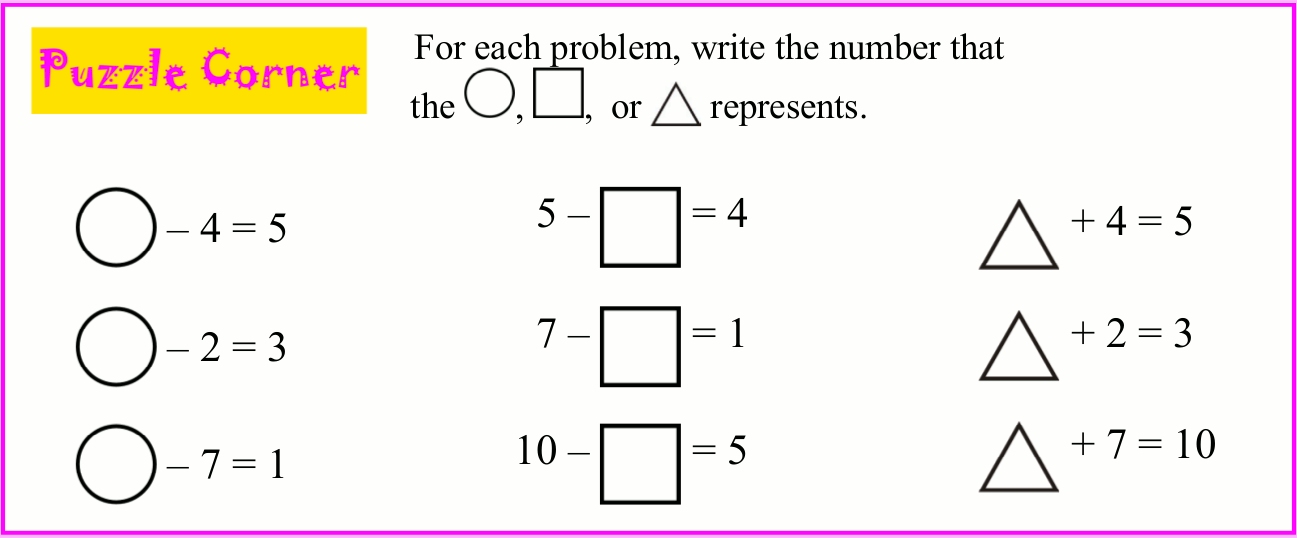# Sign changes with numbers shift from left to right of ‘ = ‘sign. Why (Additon& Subtraction)

Surekha Waldia
30-40 mins
Critical thinking
Remember, Understand, ApplicationTo understand why signs change left to right and vice versa when numbers move across = signDevelop the application of arithmetic operations
This is an important principle in arithmetic which also forms a base for bigger and important concepts like algebra
chalk , blackboard, a few balls and a bag

## Simple Addition and Subtraction A different Perspective

Step 1: You can introduce this as a puzzle activity in class and break the class into pairs so that they can brainstorm the puzzles you will be giving to them

Step 2: begin writing on the blackboard  following sum

2+5= ?

Let the kids answer the question

2+5=7 (ans)

Rewrite the same sum again:

2+ ?= 7

They wud obviously say it  aloud- 5

The catch is you need to ask them why did they arrive at 5 as the answer- in case they are unable to answer then you need to give a fresh question

Now give them a situation where numbers become objects

For example: There s a bag n which there are a total of 6 balls

As symbols write on the Blackboard-

? balls+? balls= 6 balls

Now tell them you took out two balls from the bag. Ask them where will you put the number ‘2’ on the sum written above

2 balls +? balls= 6 balls

Now ask them the next question- How many balls are left in the bag?

Answer from the children will be 4balls

2 balls +4 balls= 6 balls

Brainstorming question: What did you do to get the answer 4?

and Why did you do that operation?

Explain the logic: Now rewrite the statement as:

2balls + ?balls = 6balls

So to find out how many are left in the bag, we can minus

? balls= 6 balls -2balls

### NOTE: The emphasis has to be on the word ‘took out’ that means we are using the operation subtracting

Now give them another example with a bigger numbers

Eg: 15 balls+ ?balls =2 7balls

Give them five mins to discuss n come out with a solution. In case they are not able to get the right answer then you can go ahead explaining the logic again

1. Give them more simple sums to solve using addition sign. Once they are clear with addition sign change the sums with’ -‘ signs

Some complex examples are given below. These can be used as brain teasers and help the children solveUsing the above example you can create more sums and give children for practice and use more place values

Accurate, Complete, Knowledgeable, Precise

This s an important principle to teach coz it will develop the analytical skills of the child from the very beginning

# 1 Comment

1.Children often get confused and sometimes awed when they they see the numbers written in the form of a sum using symbols which they are not able to relate to their operations in real life . The reason being mechanical way of solving sums from a very early stage . The operation of addition should be related to increase in the given object and subtraction with decrease in the given objects . Similarly = should be understood as the conclusion of the given operation of + or _ . Once they are able to read and understand these symbols as the operation they symbolize the fear of mathematic will turn into excitement . It’s all about the way we are communicating an idea to the child .